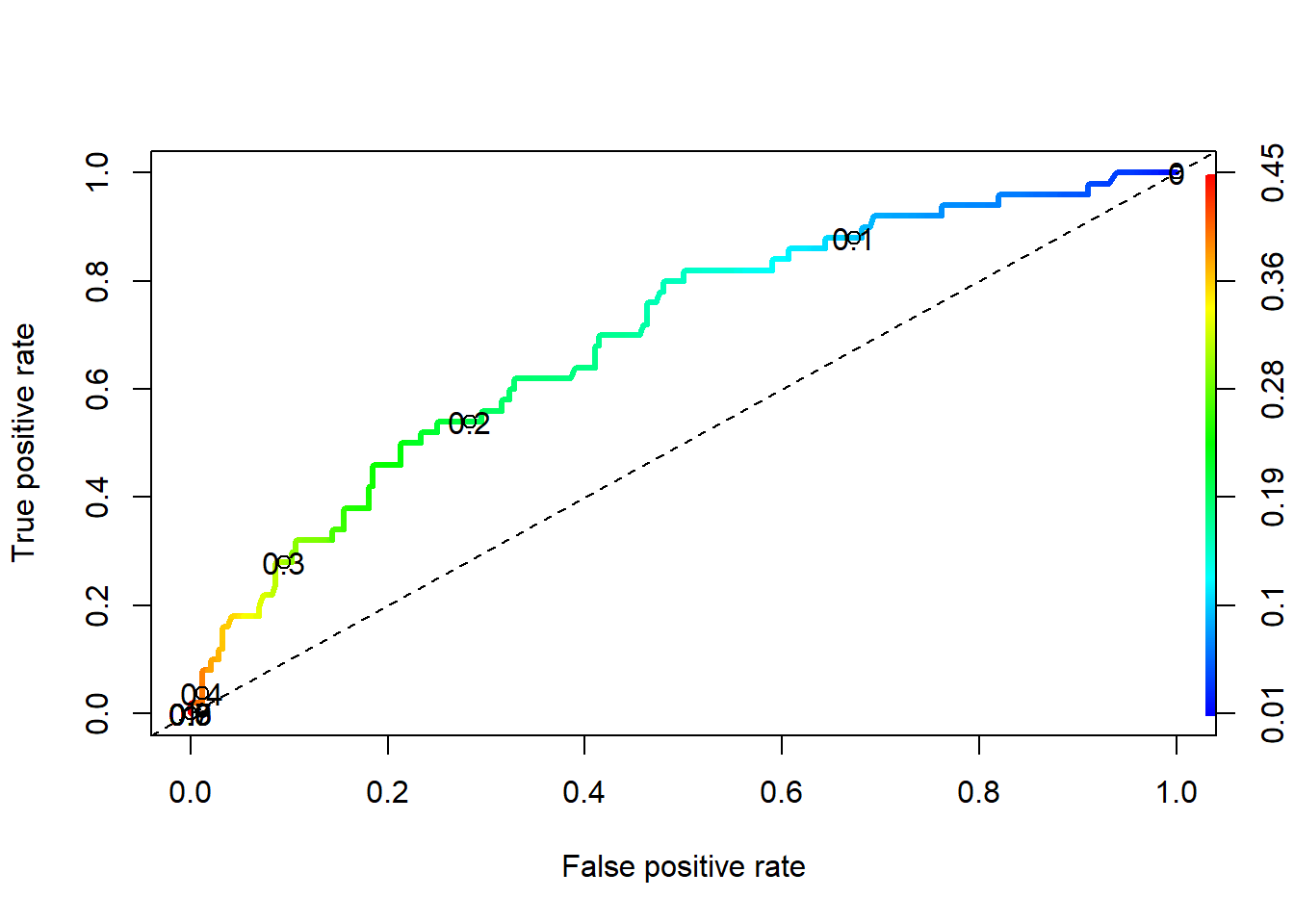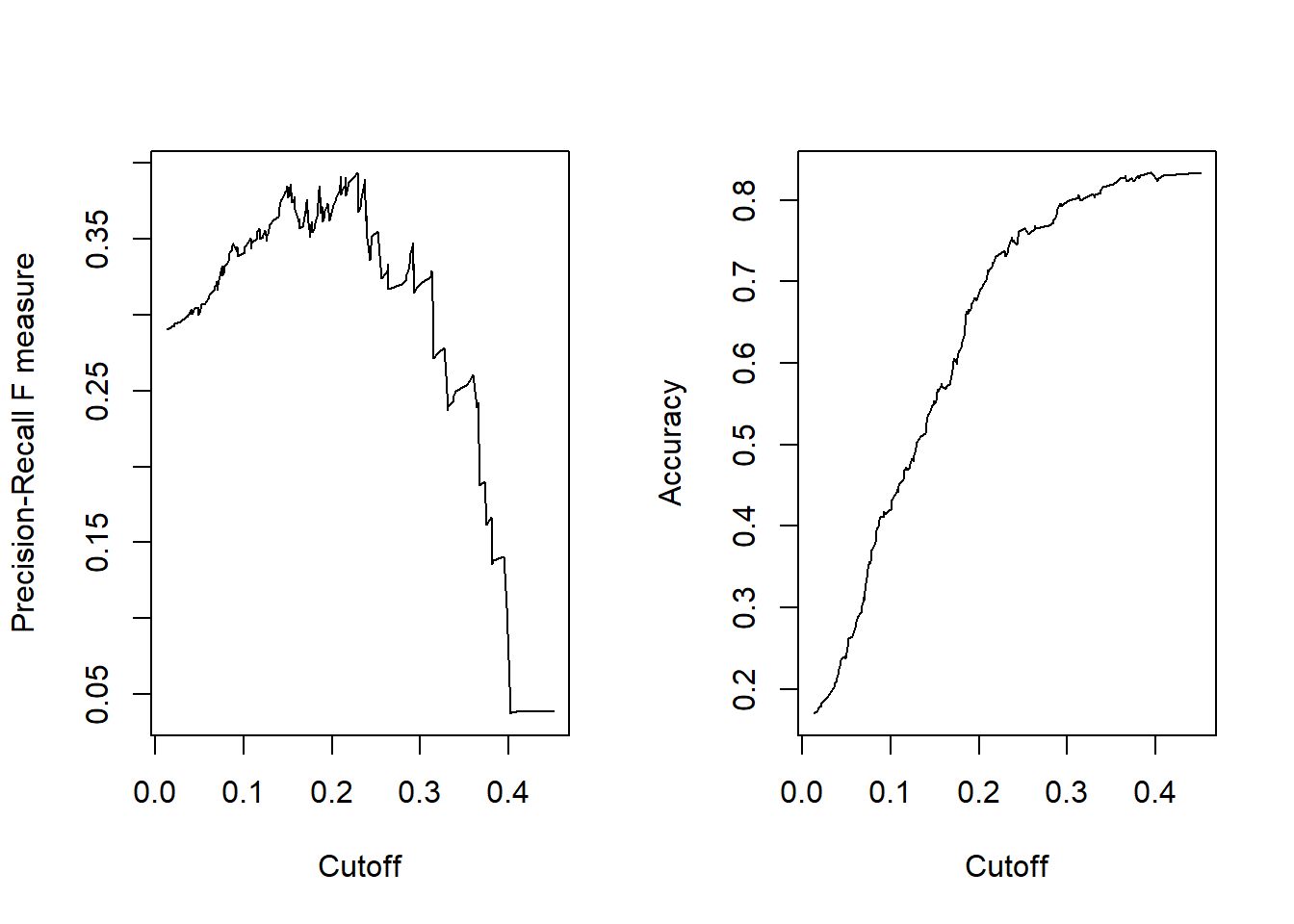## 12.6 ROC Curves

• ROC curves show the balance between sensitivity and specificity.
• We’ll use the [ROCR] package. It only takes 3 commands:
• calculate prediction() using the model
• calculate the model performance() on both true positive rate and true negative rate for a whole range of cutoff values.
• plot the curve.
• The colorize option colors the curve according to the probability cutoff point.
pr <- prediction(phat.depr, dep_sex_model\$y)
perf <- performance(pr, measure="tpr", x.measure="fpr")
plot(perf, colorize=TRUE, lwd=3, print.cutoffs.at=c(seq(0,1,by=0.1)))
abline(a=0, b=1, lty=2)We can also use the performance() function to evaluate the $$f1$$ measure

perf.f1 <- performance(pr,measure="f")
perf.acc <- performance(pr,measure="acc")

par(mfrow=c(1,2))
plot(perf.f1)
plot(perf.acc)We can dig into the perf.acc object to get the maximum accuracy value (y.value), then find the row where that value occurs, and link it to the corresponding cutoff value of x.

(max.f1 <- max(perf.acc@y.values[], na.rm=TRUE))
##  0.8333333
(row.with.max <- which(perf.acc@y.values[]==max.f1))
##  2 8
(cutoff.value <- perf.acc@x.values[][row.with.max])
##       124       256
## 0.4508171 0.3946273

Sometimes (like here) there is not one single maximum value for accuracy. In this case I would look at which of these two cutoff points maximize other metrics such as the $$f1$$ score.

ROC curves:

auc <- performance(pr, measure='auc')
auc@y.values
## []
##  0.695041